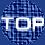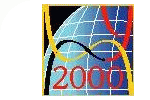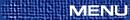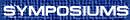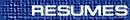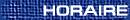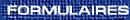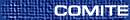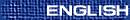Symplectic Geometry / Géométrie symplectique(Lisa Jeffrey, Organizer) MAXIM BRAVERMAN, Northeastern University, Boston, Massachusetts  02466, USA Quantization of non-compact Hamiltoninan G-spaces In the first part of the talk I will present a new regularized version of an index of a Dirac operator on a complete Riemannian manifold endowed with an action of a compact Lie group G. I show that this index is an invariant of a non-compact cobordism of the type considered by V. Guillemin, V. Ginzburg and Y. Karshon. As a corollary I obtain new proofs of the Atiyah-Segal-Singer equivariant index theorem and of the Guillemin-Sternberg ``quantization commutes with reduction'' conjecture. In the second part of the talk I'll apply the above theorems to the problem of geometric quantization of non-compact manifold. In particular, I will construct a quantization of a non-compact symplectic G-manifold. This quantization is a virtual representation of G, in which each irreducible representation appears with a finite multiplicity. ANA CANNAS DA SILVA, Instituto Superior Técnico, Lisbon, Av. Rovisco Pais, 1049-001  Lisboa, Portugal Index of contact diffeomorphisms and folded symplectic structures A folded symplectic structure is a closed 2-form which is nondegenerate except on a hypersurface, called the folding hypersurface, and whose restriction to that hypersurface has maximal rank. The folded tangent bundle is the Lie algebroid defined as the free module over smooth functions whose sections are the vector fields which are tangent to the folding hypersurface. The mapping torus of a contact diffeomorphism admits a folded symplectic structure. A contact diffeomorphism has an index defined using the folded structure on its mapping torus. Epstein and Melrose proved a formula for this index in the case of a cotangent sphere bundle of a compact manifold, which goes back to a question of Atiyah and Weinstein. This talk will report on the geometry of this index in terms of the folded tangent bundle. VIKTOR GINZBURG, University of California at Santa Cruz, Department of Mathematics, Santa Cruz, California  95064, USA Existence of periodic orbits for some classes of Hamiltonian systems We discuss the existence question for periodic orbits of Hamiltonian systems describing the motion of a charge on a Riemannian manifold and also the systems arising in semi-local generalizations of Weinstein-Moser theorem. These classes of systems are as natural and fundamental as conservative mechanical systems. However, known symplectic topology methods developed to find periodic orbits on a fixed energy level usually do not directly apply to the systems in question because the energy level may fail to have contact type. We state some new existence theorems, including results recently obtained by Ely Kerman, for periodic orbits of such Hamiltonian systems and try to explain the basic reason why periodic orbits exist for many systems of this type. REBECCA GOLDIN, Maryland A generalization of a theorem of Goresky-Kottwitz-MacPherson I Let M be a compact, connected symplectic manifold with a Hamiltonian action of a torus Tn. Define the one-skeleton of M to be the union, over all codimension 1 subtori S Ì Tn, of the fixed-point sets MS. Tolman and Weitsman proved that the equivariant cohomology of M is computable from the one-skeleton alone. Goresky, Kottwitz, and MacPherson showed that, if the one-skeleton of M has (real) dimension at most 2, then the equivariant cohomology can be computed from a combinatorial model of the one-skeleton. We generalize this GKM theorem to obtain a combinatorial computation of the equivariant cohomology of M when the one-skeleton has higher dimension. VICTOR GUILLEMIN, Department of Mathematics, MIT, Cambridge, Massachusetts  02139, USA Morse theory on graphs, part I Let G be a finite d-valent graph and G an n-dimensional torus. An ``action'' of G on G is defined by a map which assigns to each oriented edge, e, of G, a one-dimensional representation of G (or, alternatively, a weight, ae, in the weight lattice of G. For the assignment, e ® ae, to be a schematic description of a ``G-action'', these weights have to satisfy certain compatibility conditions: the GKM axioms). To this action we attach an equivariant cohomology ring, HG(G) = H(G,a). By definition this ring contains the equivariant cohomology ring of a point, S(g*) = HG(pt), as a subring, and in this talk we will use graphical versions of standard Morse theoretical techniques to analyze the structure of HG(G) as an S(g*)-module. TARA HOLM, MIT A generalization of a theorem of Goresky-Kottwitz-MacPherson II This talk continues the material covered in `A generalization of a theorem of GKM I'. JACQUES HURTUBISE, McGill University, Montreal, Quebec  H3A 2K6 Integrable systems, loop groups and surfaces Many of the most commonly studied integrable systems of mathematical physics are best understood in terms of loop algebras and loop groups, with appropriate Lie Poisson structures coming into play. It turns out that these admit separations of variables in which various ruled surfaces admitting symplectic forms come into play. LISA JEFFREY, Department of Mathematics, University of Toronto, Toronto, Ontario  M5S 3G3 Intersection pairings on singular quotients This talk describes joint work with Y.-H. Kiem, F. Kirwan and J. Woolf, in which we extend earlier results on intersection pairints on smooth symplectic quotients to the case of singular symplectic quotients. YOUNG-HOON KIEM, Yale University Intersection homology of symplectic torus quotients Let M be a Hamiltonian T-space with proper moment map m. In general, the symplectic reduction M\mathrel//T = m-1(0)/T is a compact pseudomanifold. We study the intersection (co)homology of the reduced space in terms of the equivariant cohomology H*T(m-1(0)) which is far better understood. MIKHAIL KOGAN, MIT Schubert varieties and Gelfand-Cetlin polytop Gelfand-Cetlin completely integrable system provides a set of action coordinates on every flag manifold. These coordinates map the flag manifold onto the Gelfand-Cetlin polytope. We investigate the relationship between Schubert varieties and faces of the Gelfand-Cetlin polytope. In particular, for every Schubert variety we construct a cycle in the same homology class, which is given by the preimage of some faces of the polytope. These faces can be identified with already discovered combinatorial objects (called RC-graphs). This approach provides new geometric interpretations of some formulas for double Schubert polynomials. EUGENE LERMAN, University of Illinois, Urbana, Illinois, USA Contact cuts We describe a contact analog of the symplectic cut construction As an application we show that the group of contactomorphisms for a particular overtwisted contact structure on the three sphere contains countably many nonconjugate two tori. STEVEN LILLYWHITE, University of Toronto, Toronto, Ontario  M5S 3G3 The topology of symplectic reductions of loop spaces We compute the real homotopy type of a symplectic reduction of the loop space of the 2-sphere by SO(3). This symplectic reduction may be identified with a moduli space of arc-length parametrised closed curves in Euclidean space. In this case, the Kirwan map turns out to be an isomorphism. We discuss how to compute the equivariant cohomology of loop spaces. EYAL MARKMAN, University of Massachusetts, Amherst, Massachusetts  01003, USA Hyperkahler varieties; their reflections and monodromy Reflections of holomorphic symplectic varieties are certain symplectic birational (surgery) operations. They play a central role in the study of K3 surfaces and higher dimensional holomorphic symplectic varieties. 1)  We construct holomorphic-symplectic reflections modeled after dual pairs of Springer resolutions of nilpotent coadjoint orbits. 2)  We derive a Picard-Lefschetz formula, describing the reflection on the level of cohomology rings. 3)  We apply these results to study the monodromy representation on the cohomology ring of Hilbert scheme of points on a K3 surface (and other moduli spaces of vector bundles on a K3). REYER SJAMAAR, Department of Mathematics, Cornell University, Ithaca, New York  14853-4201, USA Moment maps and Riemannian symmetric pairs This is joint work with Luis O'Shea. Our main result is a ``real form'' of Kirwan's convexity theorem. We apply our result to flag varieties of real semisimple groups and thus obtain eigenvalue inequalities, which generalize inequalities found by Weyl, Ky Fan, Kostant, Klyachko, and many others. For instance, we find an answer to the following question: given the singular values of two rectangular matrices (of the same size), what are the possible singular values of their sum? JENNIFER SLIMOWITZ, Rice University, Houston, Texas, USA Shortening the Hofer length of Hamiltonian circle actions Consider a Hamiltonian circle action on a symplectic manifold (M,w). If the moment map of this action obtains its maximum at an isolated fixed point at which one of the weights has absolute value greater than one, then this circle action may be deformed to a loop in Hamc(M) with shorter Hofer length. Following ideas of Polterovich, we produce shorter loops and describe an estimate for the amount by which the original loop may be shortened. MARGARET SYMINGTON, Texas-Austin Singular Lagrangian fibrations in dimension four We study the class of all closed symplectic 4-manifolds M that admit a smooth map to a two dimensional base that, over an open dense subset U of the base, give the manifold the structure of a Lagrangian fibration. The preimage of a point in the complement of U may be a singular fiber of a restricted type. The set of allowable singular fibers includes lower dimensional tori (as appear in a toric variety) and nodal fibers (which appear in holomorphic fibrations and a Lagrangian fibration of the K3 surface). SUE TOLMAN, University of Illinois at Urbana-Champaign, Urbana, Illinois  61801-2975, USA Symplectic packing, Gromov width, and Grassmanians Joint work with Y. Karshon. As an application of our project to classify complexity one spaces, we find a symplectic packing of several Grassmanians by two balls, and hence obtain an lower bound on the Gromov Width. LISA TRAYNOR, Bryn Mawr College Invariants for legendrian tangles A recent development has been the construction of legendrian knot invariants from contact homology. This standard contact homology is defined using holomorphic curves in the symplectization of a contact manifold. For legendrian tangles, there is an alternative definition for contact homology coming from the theory of generating functions. I will compare and contrast these notions of contact homology. THOMAS TRELOAR, Department of Mathematics, University of Maryland, College Park, Maryland  20742, USA Manin pairs and the symplectic geometry of polygons We obtain a symplectic structure on the moduli space of closed n-gons with fixed side-lengths in the 3-sphere. This structure arises from a quasi-hamiltonian reduction on the fusion product of n-copies of S3, where S3 is equipped with a quasi-poisson bivector induced from Manin pair (su2 Åsu2, su2). This is a completely integrable system and the hamiltonian flows can be calculated. More generally, the symplectic structure on the moduli space of n-gons in any constant curvature 3-space can be given as a reduction associated to a Manin pair (d,g) where g = su2. These structures relate to the structures obtained from the moduli space of flat connections on a n-punctured 2-sphere. JONATHAN WEITSMAN, California-Santa Cruz To be announced CATALIN ZARA, Department of Mathematics, MIT, Cambridge, Massachusetts  02139, USA Morse theory on graphs, part II Continuation of the previous talk.